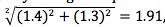## Monday, August 31, 2015

### Statistic Problems

A Statistics Problem With Implications for Montana Mathematics
by David Erickson, University of Montana

As far back as the 1960s, the National Assessment of Educational  Progress (NAEP) nicknamed the nation’s report card began taking a snapshot on the nation’s students’ progress in mathematics. Fourth and eighth graders are tested systematically across the country and test results can be compared by states and the nation. For Montana, in 2013, 8th grade teacher’s mathematics background (mathematics education major vs. no mathematics education major) was recorded along with his/her students’ average scale scores on the test.  Eighth grade Montana teachers with a mathematics education major had students average scale scores of 294 with a standard error of 1.4, whereas those teachers with no mathematics education major students scored 285 with a standard error of 1.3.

 8th Grade NAEP by Teacher Education Math Ed Major No Math Ed Major Year Jurisdiction Average scale score Standard error Average scale score Standard error 2013 National public 288 (0.6) 281 (0.4) Montana 294 (1.4) 285 (1.3)

One interesting question would be, are these scores different and if so, how different?

We know that the average scale score difference in Montana is 9 points (294-285). We can calculate the standard error difference of these two scale scores by taking the square root of  the sum of the squares of the individual standard errors, soor a SE on the difference of 1.91.  To calculate the relative size of that over the 9 point difference, we divide 9 by 1.91 to obtain 4.71. A difference of 2 standard deviations would contain a confidence interval of about 95% of the data, and 3 standard deviations of about 99.7%, but this translates into almost a 1 in a million chance of obtaining a scale score of this difference due to chance alone.   So, the scores are different, very different.

Unfortunately, we have only a correlation, not a causation.  We know mathematics content knowledge matters and so it is tempting to assume that is the cause of the difference in scores, but it could be that the teachers with mathematics education majors were employed in districts that had better mathematics students and thus, the students in those districts were different from students in other districts, and the cause was not the teachers knowledge/background alone, but what district they taught in.  We don’t have access to that data from NAEP.

What other questions should we be asking?  What implications for Montana mathematics education are there?  Send me your comments/concerns/suggestions/questions. david.erickson@umontana.edu